In :
import warnings
warnings.filterwarnings('ignore')
warnings.simplefilter('ignore')

In :
%matplotlib notebook
import paramonte as pm
import numpy as np
import scipy as sp
import pandas as pd
import seaborn as sns
import matplotlib as mpl
import matplotlib.pyplot as plt
print('\n'.join(f'{m.__name__} {m.__version__}' for m in globals().values() if getattr(m, '__version__', None)))
sns.set()

paramonte 2.5.1
numpy 1.19.5
scipy 1.5.4
pandas 1.2.0
seaborn 0.11.0
matplotlib 3.3.3


## Sampling a 4-dimensional MultiVariate Normal distribution (MVN) via the ParaMonte library's ParaDRAM routine¶

The logic of Monte Carlo sampling is very simple:

Suppose we have a mathematical objective function defined on a ndim-dimensional domain. Typically, this domain is defined on the space of real numbers. In general, understanding and visualizing the structure of such objective functions is an extremely difficult task, if not impossible. As a better alternative, you employ Monte Carlo techniques that can randomly explore the structure of the objective function and find the function's extrema.

In the following example below, an example of one of the simplest such objective functions, the Multivariate Normal Distribution (MVN), is constructed and sampled using the ParaMonte library samplers, here, the ParaDRAM sampler (Delayed-Rejection Adaptive Metropolis-Hastings Markov Chain Monte Carlo sampler).

Suppose we want to sample random points from a MultiVariate Normal (MVN) Probability Density Function (PDF). The PDF equation of MVN is the following,

$$\large \pi (x, \mu, \Sigma, k = 4) = (2\pi)^{-\frac{k}{2}} ~ |\Sigma|^{-\frac{1}{2}} ~ \exp \bigg( -\frac{1}{2} (x-\mu)^T ~ \Sigma^{-1} ~ (x-\mu) \bigg)$$

The following Python function getLogFunc() returns the natural logarithm of the Probability Density Function of the multivariate Normal distribution.

Since the mathematical objective functions (e.g., probability density functions) can take extremely small or large values, we often work with their natural logarithms instead. This is the reason behind the naming convention used in the ParaMonte library for the user's objective functions: getLogFunc, implying that the user must provide a function that returns the natural logarithm of the target objective function.

See this Jupyter Notebook for an in-depth discussion of why we need to work with the logarithm of mathematical objective functions in optimization and sampling problems.

In :
import numpy as np

NDIM = 4                                # number of dimensions of the domain of the MVN PDF
MEAN =  np.double([-10, 15., 20., 0.0]) # This is the mean of the MVN PDF.
COVMAT = np.double( [ [1.0,.45,-.3,0.0] # This is the covariance matrix of the MVN PDF.
, [.45,1.0,0.3,-.2]
, [-.3,0.3,1.0,0.6]
, [0.0,-.2,0.6,1.0]
] )

INVCOV = np.linalg.inv(COVMAT) # This is the inverse of the covariance matrix of the MVN distribution.

# The following is the log of the coefficient used in the definition of the MVN.

MVN_COEF = NDIM * np.log( 1. / np.sqrt(2.*np.pi) ) + np.log( np.sqrt(np.linalg.det(INVCOV)) )

# the logarithm of objective function: log(MVN)

def getLogFunc(point):
"""
Return the logarithm of the MVN PDF.
"""
normedPoint = MEAN - point
return MVN_COEF - 0.5 * ( np.dot(normedPoint,np.matmul(INVCOV,normedPoint)) )


We will sample random points from the objective function by calling the ParaDRAM sampler (Delayed-Rejection Adaptive Metropolis-Hastings Markov Chain Monte Carlo sampler) of the ParaMonte library.

## Running a ParaDRAM simulation on a single processor¶

To run the sampler in parallel, you will have to first save the MPI-enabled script as an external file. Visit the ParaMonte library's documentation website for more information, or see this parallel ParaDRAM simulation Jupyter notebook example.

In :
import paramonte as pm


First we will check what version of the ParaMonte library your are using,

In :
print( pm.version.kernel.get() )
print( pm.version.interface.get() )

ParaMonte Python Kernel Version 1.5.1
ParaMonte Python Interface Version 2.5.1


We can also dump the ParaMonte library interface version,

In :
print( pm.version.kernel.dump() )
print( pm.version.interface.dump() )

1.5.1
2.5.1


Checking for the new versions of the ParaMonte library is also easy and recommended,

In :
pm.checkForUpdate()

ParaMonte - NOTE: You have the latest version of the ParaMonte library.
ParaMonte - NOTE: To see the most recent changes to the library, visit,
ParaMonte - NOTE:
ParaMonte - NOTE:     https://www.cdslab.org/paramonte/notes/overview/paramonte-python-release-notes



We can also verify the existence of the essential components of the current installation of the ParaMonte Python library on the system,

In :
pm.verify()

:::::::::::::::::::::::::::::::::::::::::::::::::::::::::::::::::::::::::::::::::::::::::::::::
::::                                                                                       ::::

_/_/_/_/                                   _/_/    _/_/
_/    _/                                  _/_/_/_/_/                     _/
_/    _/ _/_/_/_/   _/ /_/_/ _/_/_/_/     _/  _/  _/   _/_/   _/_/_/   _/_/_/  _/_/_/
_/_/_/   _/    _/   _/_/     _/    _/     _/      _/  _/   _/ _/    _/   _/   _/_/_/_/
_/       _/    _/   _/       _/    _/     _/      _/  _/   _/ _/    _/   _/   _/
_/_/_/       _/_/_/_/ _/         _/_/_/_/ _/_/_/  _/_/_/  _/_/  _/    _/   _/_/   _/_/_/

ParaMonte
plain powerful parallel
Monte Carlo library
Version 2.5.1

::::                                                                                       ::::
:::::::::::::::::::::::::::::::::::::::::::::::::::::::::::::::::::::::::::::::::::::::::::::::

ParaMonte - NOTE: The ParaMonte::Kernel samplers have no Python package dependencies
ParaMonte - NOTE: beyond numpy. However, the ParaMonte::Python post-processing and
ParaMonte - NOTE: visualization tools require the following Python packages,
ParaMonte - NOTE:
ParaMonte - NOTE:     numpy : 1.19.2
ParaMonte - NOTE:     scipy : 1.5.2
ParaMonte - NOTE:     pandas : 1.1.2
ParaMonte - NOTE:     seaborn : 0.11.0
ParaMonte - NOTE:     matplotlib : 3.3.2
ParaMonte - NOTE:
ParaMonte - NOTE: If you do not intend to use the postprocessing and visualization tools,
ParaMonte - NOTE: you can ignore this message. Otherwise,
ParaMonte - NOTE:
ParaMonte - NOTE:     UPDATE THE ABOVE PACKAGES TO THE REQUESTED VERSIONS OR NEWER TO ENABLE
ParaMonte - NOTE:     THE VISUALIZATION AND POSTPROCESSING TOOLS OF THE ParaMonte LIBRARY.

ParaMonte - NOTE: Intel MPI library for 64-bit architecture detected at:
ParaMonte - NOTE:
ParaMonte - NOTE:     C:\Program Files (x86)\IntelSWTools\compilers_and_libraries_2020.1.216\windows\mpi\intel64\bin
ParaMonte - NOTE:
ParaMonte - NOTE: To perform ParaMonte simulations in parallel on a single node,
ParaMonte - NOTE: run the following two commands, in the form and order specified,
ParaMonte - NOTE: on a Python-aware mpiexec-aware command-line interface such as
ParaMonte - NOTE: Anaconda3 Windows command prompt:
ParaMonte - NOTE:
ParaMonte - NOTE:     "C:\Program Files (x86)\IntelSWTools\compilers_and_libraries_2020.1.216\windows\mpi\intel64\bin\mpivars.bat"
ParaMonte - NOTE:
ParaMonte - NOTE:     mpiexec -localonly -n 2 python main_mpi.py
ParaMonte - NOTE:
ParaMonte - NOTE: where,
ParaMonte - NOTE:
ParaMonte - NOTE:     0.   the first command defines the essential environment variables,
ParaMonte - NOTE:          and the second command runs in the simulation in parallel, where,
ParaMonte - NOTE:     1.   you should replace the number 2 with the desired processor count
ParaMonte - NOTE:          you wish to assign to your simulation task and,
ParaMonte - NOTE:     2.   the flag '-localonly' indicates a parallel simulation on only
ParaMonte - NOTE:          a single node (this flag will obviate the need for the MPI
ParaMonte - NOTE:          https://software.intel.com/en-us/get-started-with-mpi-for-windows
ParaMonte - NOTE:     3.   python is the name of the Python software installed on your system.
ParaMonte - NOTE:          If python is not recognized on your command line, use python3 instead.
ParaMonte - NOTE:     4.   main_mpi.py is the Python file which serves as the entry point to
ParaMonte - NOTE:          your simulation, where you call the ParaMonte sampler routines.
ParaMonte - NOTE:
ParaMonte - NOTE: Note that the above two commands must be executed on a command-line that
ParaMonte - NOTE: recognizes both Python and mpiexec applications, such as the Anaconda
ParaMonte - NOTE: command-line interface. For more information, in particular, on how
ParaMonte - NOTE: to register to run Hydra services for multi-node simulations
ParaMonte - NOTE: on Windows servers, visit:
ParaMonte - NOTE:
ParaMonte - NOTE:     https://www.cdslab.org/paramonte

ParaMonte - NOTE: To check for the MPI library installation status or display the above
ParaMonte - NOTE: messages in the future, type the following on the Python command-line:
ParaMonte - NOTE:
ParaMonte - NOTE:     import paramonte as pm
ParaMonte - NOTE:     pm.verify()
ParaMonte - NOTE:
ParaMonte - NOTE: To get started, type the following on the Python command-line,
ParaMonte - NOTE:
ParaMonte - NOTE:     import paramonte as pm
ParaMonte - NOTE:     pm.helpme()
ParaMonte - NOTE:
ParaMonte - NOTE: To get help on individual components of the paramonte modules,
ParaMonte - NOTE: pass the name of the components to helpme(). For example,
ParaMonte - NOTE:
ParaMonte - NOTE:     import paramonte as pm



### Setting up the ParaDRAM simulation specifications¶

Now, define a ParaDRAM sampler instance,

In :
pmpd = pm.ParaDRAM()


The simplest scenario is to run the simulation with the default specifications that are appropriately determined by the ParaDRAM sampler. However, for the purpose of clarity reproducibility, we will specify a few simulation specs,

For a complete list of all ParaDRAM simulation specifications, visit the ParaDRAM simulation specifications webpage.

### The simulation output file names and storage path¶

We will store all output files in a directory with the same name as this Jupyter Notebook's name and with the same prefix.

By default, all ParaDRAM simulation output files are named properly (see this page for a review of ParaDRAM simulation output files). In general, it is a good habit to specify an output file prefix for any ParaDRAM simulation. This way, the restart of an incomplete simulation, if it happens for some reasons, would be seamless and doable by just rerunning the simulation, without any change of any sort to any parts of the codes or the simulation.

In :
pmpd.spec.outputFileName = "./out/mvn_serial"


By default, the ParaMonte samplers do not overwrite old existing simulation files for obvious reasons. For this example, however, we will let the sampler overwrite the simulation output files if they already exist at the specified path,

In :
pmpd.spec.overwriteRequested = True


### Ensuring the reproducibility of the results¶

We will also initialize the random seed to a fixed number to ensure the reproducibility of the simulation results in the future,

In :
pmpd.spec.randomSeed = 3751


Not so important, but we will also specify a chainSize here, 30000, the number of unique points to be sampled from the objective function. This number is much smaller than the default 100,000 unique sample points of the sampler.

In :
pmpd.spec.chainSize = 30000


For the sake of illustration, we will also request an ASCII-format restart output file,

In :
pmpd.spec.restartFileFormat = "ascii"


Note that none of the above specification settings are necessary, but is in general a good habit to define them prior to the simulation.
We now call the ParaDRAM sampler routine to run the sampling simulation,

In :
# run the ParaDRAM sampler
pmpd.runSampler ( ndim = 4
, getLogFunc = getLogFunc
)

ParaDRAM - NOTE: Running the ParaDRAM sampler in serial mode...
ParaDRAM - NOTE: To run the ParaDRAM sampler in parallel mode visit:
ParaDRAM - NOTE: If you are using Jupyter notebook, check the Jupyter's
ParaDRAM - NOTE: terminal window for realtime simulation progress and report.

ParaDRAM - NOTE: where you should replace pmpd with your ParaDRAM sampler's object name.



This will print the realtime simulation progress information on your Anaconda prompt window (not inside your Jupyter notebook). Once the simulation is finished, the ParaDRAM routine generates 5 output files, each of which contains information about certain aspects of the simulation,

• mvn_serial_process_1_report.txt
This file contains reports about all aspects of the simulation, from the very beginning of the simulation to the very last step, including the postprocessing of the results and final sample refinement.

• mvn_serial_process_1_progress.txt
This file contains dynamic realtime information about the simulation progress. The frequency of the progress report to this file depends on the value of the simulation specification progressReportPeriod.

• mvn_serial_process_1_sample.txt
This file contains the final fully-refined decorrelated i.i.d. random sample from the objective function.

• mvn_serial_process_1_chain.txt
This file contains the Markov chain, generally in either binary or condensed (compact) ASCII format to improve the storage efficiency of the chain on your computer.

Now, we can read the generated output sample, contained in the file suffixed with *_sample.txt.

In :
pmpd.readSample() # generates pmpd.sampleList
sample = pmpd.sampleList

ParaDRAM - WARNING: The delimiter is neither given as input to readSample()
ParaDRAM - WARNING: nor set as a simulation specification of the ParaDRAM object.
ParaDRAM - WARNING: This information is essential, otherwise how could the output files be parsed?
ParaDRAM - WARNING: For now, the ParaDRAM sampler will assume a comma-separated
ParaDRAM - WARNING: file format for the contents of the sample file(s) to be parsed.

ParaDRAM - NOTE: 1 files detected matching the pattern: "./out/mvn_serial*_sample.txt"

ParaDRAM - NOTE: ndim = 4, count = 8924
ParaDRAM - NOTE: parsing file contents...
ParaDRAM - NOTE: computing the sample correlation matrix...
ParaDRAM - NOTE: creating a heatmap plot object from scratch... done in 0.020009 seconds.
ParaDRAM - NOTE: computing the sample covariance matrix...
ParaDRAM - NOTE: creating a heatmap plot object from scratch... done in 0.026999 seconds.
ParaDRAM - NOTE: computing the sample autocorrelations...
ParaDRAM - NOTE: creating a line plot object from scratch... done in 0.0 seconds.
ParaDRAM - NOTE: creating a scatter plot object from scratch... done in 0.0 seconds.
ParaDRAM - NOTE: creating a lineScatter plot object from scratch... done in 0.0 seconds.
ParaDRAM - NOTE: creating a line plot object from scratch... done in 0.0 seconds.
ParaDRAM - NOTE: creating a scatter plot object from scratch... done in 0.0 seconds.
ParaDRAM - NOTE: creating a lineScatter plot object from scratch... done in 0.0 seconds.
ParaDRAM - NOTE: creating a line3 plot object from scratch... done in 0.000989 seconds.
ParaDRAM - NOTE: creating a scatter3 plot object from scratch... done in 0.0 seconds.
ParaDRAM - NOTE: creating a lineScatter3 plot object from scratch... done in 0.0 seconds.
ParaDRAM - NOTE: creating a jointplot plot object from scratch... done in 0.0 seconds.
ParaDRAM - NOTE: creating a histplot plot object from scratch... done in 0.0 seconds.
ParaDRAM - NOTE: creating a kdeplot1 plot object from scratch... done in 0.0 seconds.
ParaDRAM - NOTE: creating a kdeplot2 plot object from scratch... done in 0.0 seconds.
ParaDRAM - NOTE: creating a contour3 plot object from scratch... done in 0.0 seconds.
ParaDRAM - NOTE: creating a contourf plot object from scratch... done in 0.0 seconds.
ParaDRAM - NOTE: creating a contour plot object from scratch... done in 0.0 seconds.
ParaDRAM - NOTE: creating a grid plot object from scratch... done in 0.001002 seconds.

ParaDRAM - NOTE: The processed sample files are now stored in the newly-created
ParaDRAM - NOTE: component sampleList of the ParaDRAM object as a Python list.
ParaDRAM - NOTE: For example, to access the contents of the first (or the only) sample file, try:
ParaDRAM - NOTE: where you will have to replace pmpd with your ParaDRAM instance name.
ParaDRAM - NOTE: To access the plotting tools, try:
ParaDRAM - NOTE:     pmpd.sampleList.plot.<PRESS TAB TO SEE THE LIST OF PLOTS>
ParaDRAM - NOTE:     pmpd.sampleList.plot.line()         # to make 2D line plots.
ParaDRAM - NOTE:     pmpd.sampleList.plot.scatter()      # to make 2D scatter plots.
ParaDRAM - NOTE:     pmpd.sampleList.plot.lineScatter()  # to make 2D line-scatter plots.
ParaDRAM - NOTE:     pmpd.sampleList.plot.line3()        # to make 3D line plots.
ParaDRAM - NOTE:     pmpd.sampleList.plot.scatter3()     # to make 3D scatter plots.
ParaDRAM - NOTE:     pmpd.sampleList.plot.lineScatter3() # to make 3D line-scatter plots.
ParaDRAM - NOTE:     pmpd.sampleList.plot.contour()      # to make fast 2D kernel density plots.
ParaDRAM - NOTE:     pmpd.sampleList.plot.contourf()     # to make fast 2D kernel density filled contour plots.
ParaDRAM - NOTE:     pmpd.sampleList.plot.contour3()     # to make fast 3D kernel density contour plots.
ParaDRAM - NOTE:     pmpd.sampleList.plot.histplot()     # to make seaborn 1D distribution plots.
ParaDRAM - NOTE:     pmpd.sampleList.plot.kdeplot1()     # to make seaborn 1D kernel density plots.
ParaDRAM - NOTE:     pmpd.sampleList.plot.kdeplot2()     # to make seaborn 2D kernel density plots.
ParaDRAM - NOTE:     pmpd.sampleList.plot.grid()         # to make GridPlot
ParaDRAM - NOTE: To plot or inspect the variable autocorrelations or the correlation/covariance matrices, try:
ParaDRAM - NOTE:     pmpd.sampleList.stats.<PRESS TAB TO SEE THE LIST OF COMPONENTS>



By default, the sampler generates a highly refined decorrelated final sample. Essentially, the ParaDRAM sampler refining the Markov chain for as long as there is any residual auto-correlation in any of the individual variables of the Markov chain that has been sampled. This is an extremely strong and conservative refinement strategy (and it is done so in the ParaDRAM algorithm for a good reason: to ensure independent and identically distributed (i.i.d.) samples from the objective function.

This extreme refinement can be relaxed and controlled by the user, by setting the following ParaDRAM simulation specification variable prior to the simulation run,

In :
pmpd.spec.sampleRefinementCount = 1


If you rerun the simulation with this specification set and, you will notice about a three-fold increase in the final sample size. Note that the above specification has to be set before running the simulation in the above if you really want to use it (also, do not forget to specify a new output file prefix for the new simulation because simulations cannot be overwritten). A value of 1 will cause the ParaDRAM sampler to refine the Markov chain for only one round, which what most people in the MCMC community do.

To quickly visualize the generated sample as a histogram, try,

In :
sample.plot.histplot()

ParaDRAM - NOTE: making the histplot plot...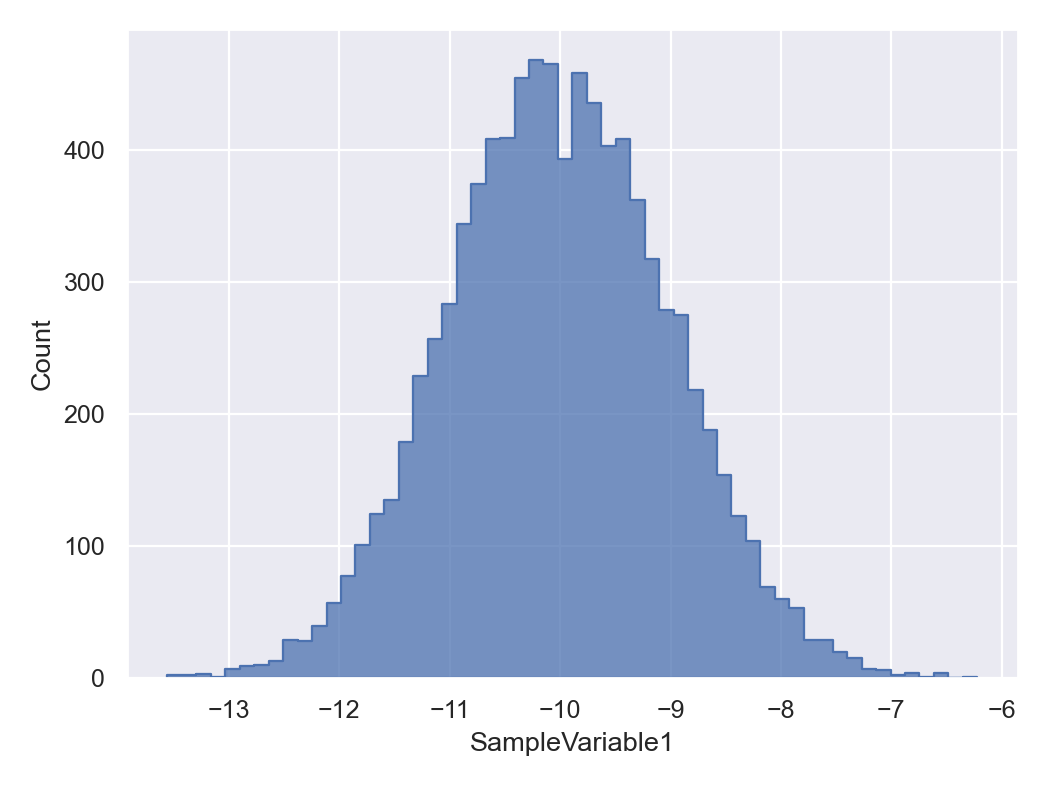done in 0.147 seconds.


How about adding a target value to this histogram plot? By default, if no value is specified for the target object, it will add the state corresponding to the mode of objective funciton to the plot, as inferred from the sample file,

In :
sample.plot.histplot.reset()
sample.plot.histplot()
sample.plot.histplot.currentFig.axes.set_xlabel("Standard Gaussian Random Value")
sample.plot.histplot.target() # add a line corresponding to the maxLogFunc (mode) of the sampled points.

ParaDRAM - NOTE: making the histplot plot...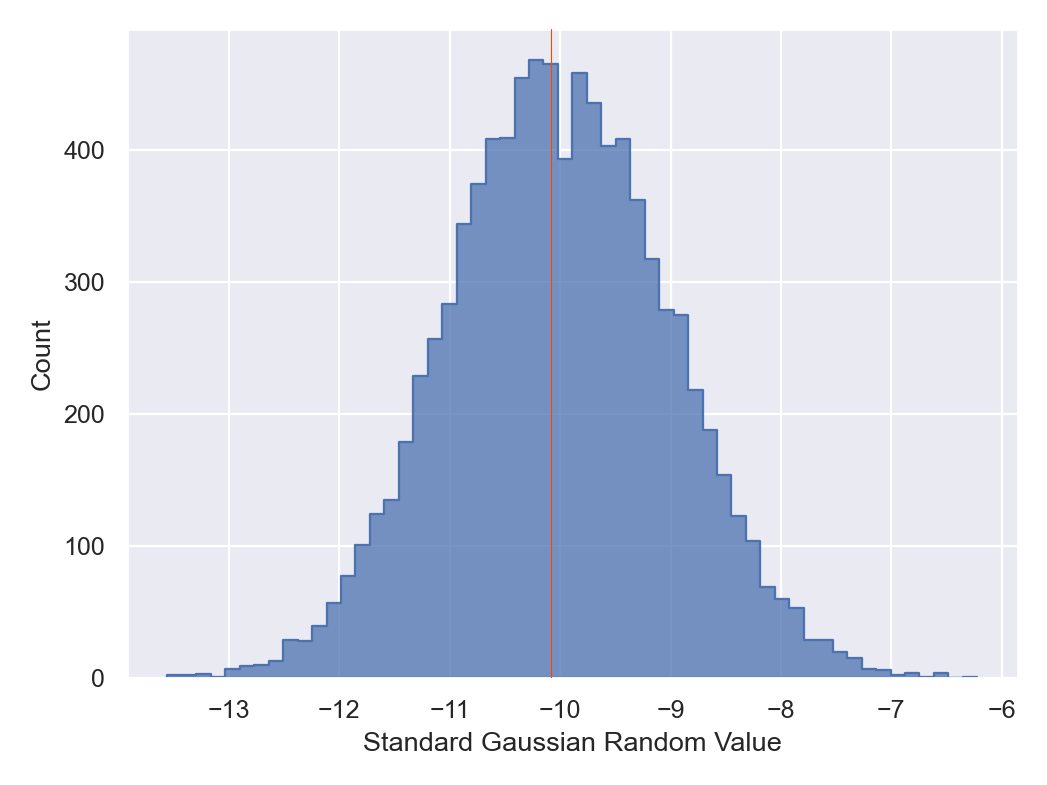done in 0.111 seconds.


However, assiging other values is also possible. For example, let's say that we want to add the mean and 1-sigma limits to the plot, instead of the single mode,

In :
sample.plot.histplot()
sample.plot.histplot.currentFig.axes.set_xlabel("Standard Gaussian Random Value")

avg = sample.df.SampleVariable1.mean()
sample.plot.histplot.target( value = avg )

std = sample.df.SampleVariable1.std()
sample.plot.histplot.target.axvline.kws.linestyle = "--"
sample.plot.histplot.target( value = avg - std )
sample.plot.histplot.target( value = avg + std )

ParaDRAM - NOTE: making the histplot plot...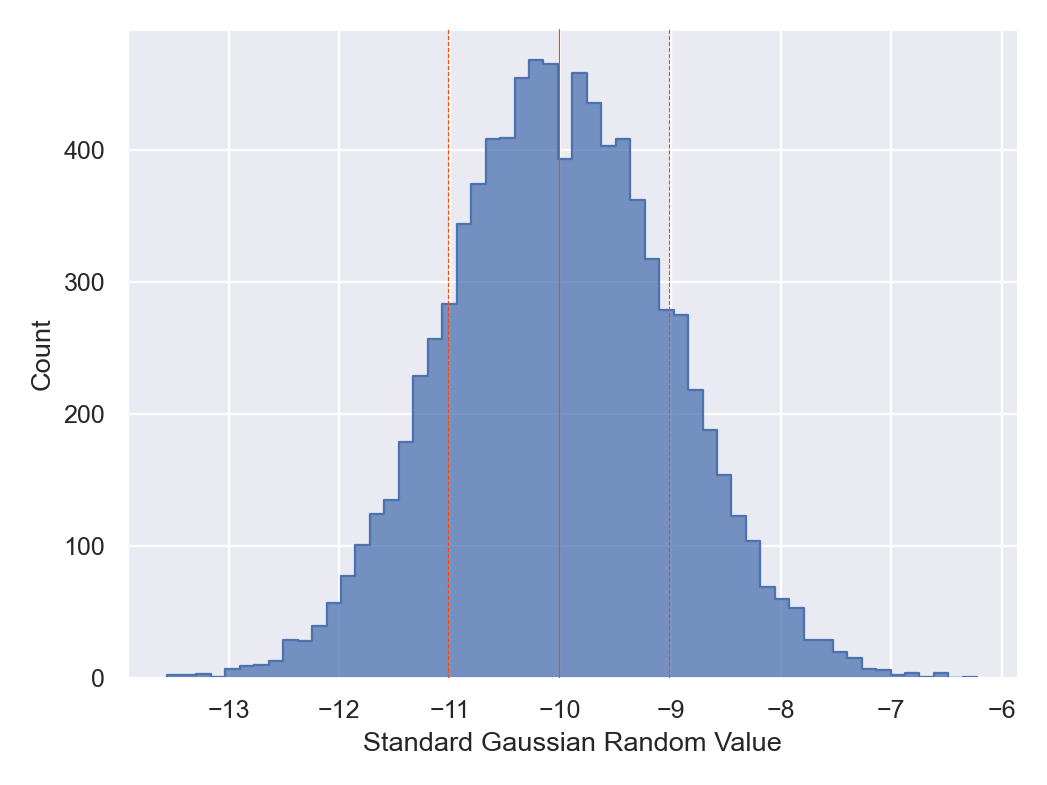done in 0.109998 seconds.


We can also plot all of the variables at the same time in a single figure,

In :
sample.df.head()

Out:
SampleLogFunc SampleVariable1 SampleVariable2 SampleVariable3 SampleVariable4
0 -7.437893 -10.602866 13.914840 21.541231 1.239213
1 -7.437893 -10.602866 13.914840 21.541231 1.239213
2 -6.866939 -11.305132 14.502793 22.145923 1.054045
3 -4.833832 -11.356071 13.499396 20.976090 1.235661
4 -4.833832 -11.356071 13.499396 20.976090 1.235661
In :
sample.plot.histplot( xcolumns = [1,2,3,4] )

ParaDRAM - NOTE: making the histplot plot...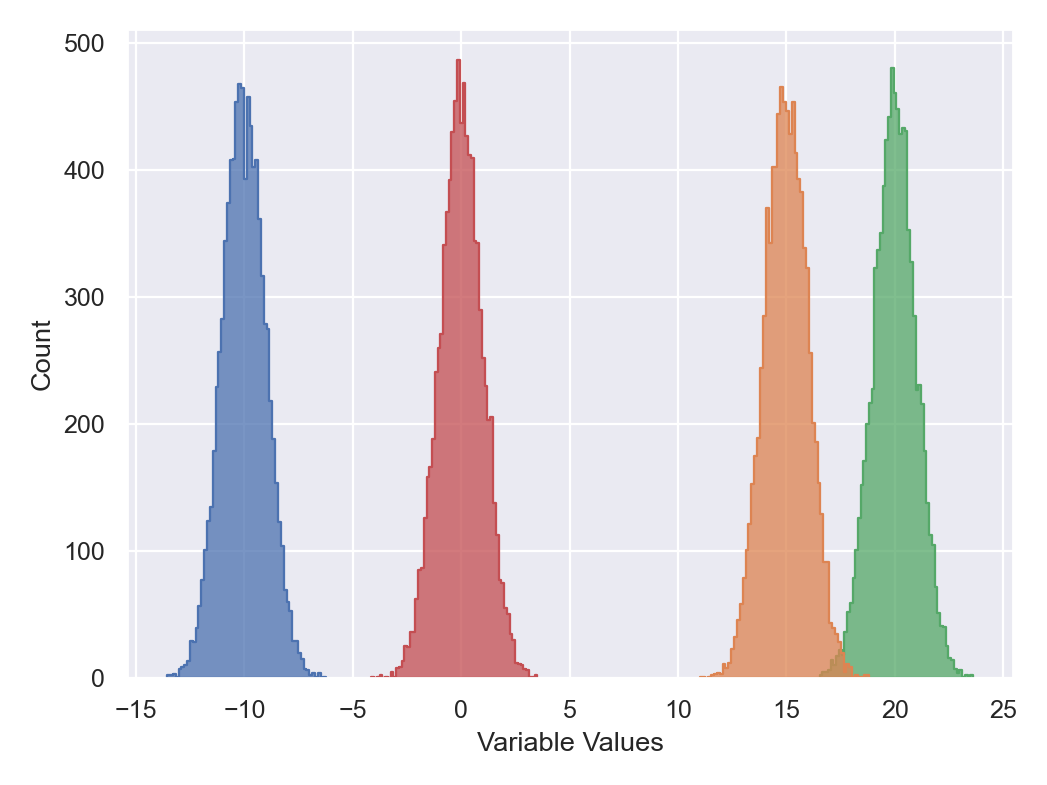done in 0.246995 seconds.


Perhaps, we would also want to add legend and change the x-axis title,

In :
sample.plot.histplot.reset("hard") # reseting to the default is not necessary, but does not hurt either.
sample.plot.histplot.legend.enabled = True
sample.plot.histplot( xcolumns = [1,2,3,4] )
sample.plot.histplot.currentFig.axes.set_xlabel("Multivariate Normal Variables")

ParaDRAM - NOTE: creating a histplot plot object from scratch... done in 0.0 seconds.
ParaDRAM - NOTE: making the histplot plot...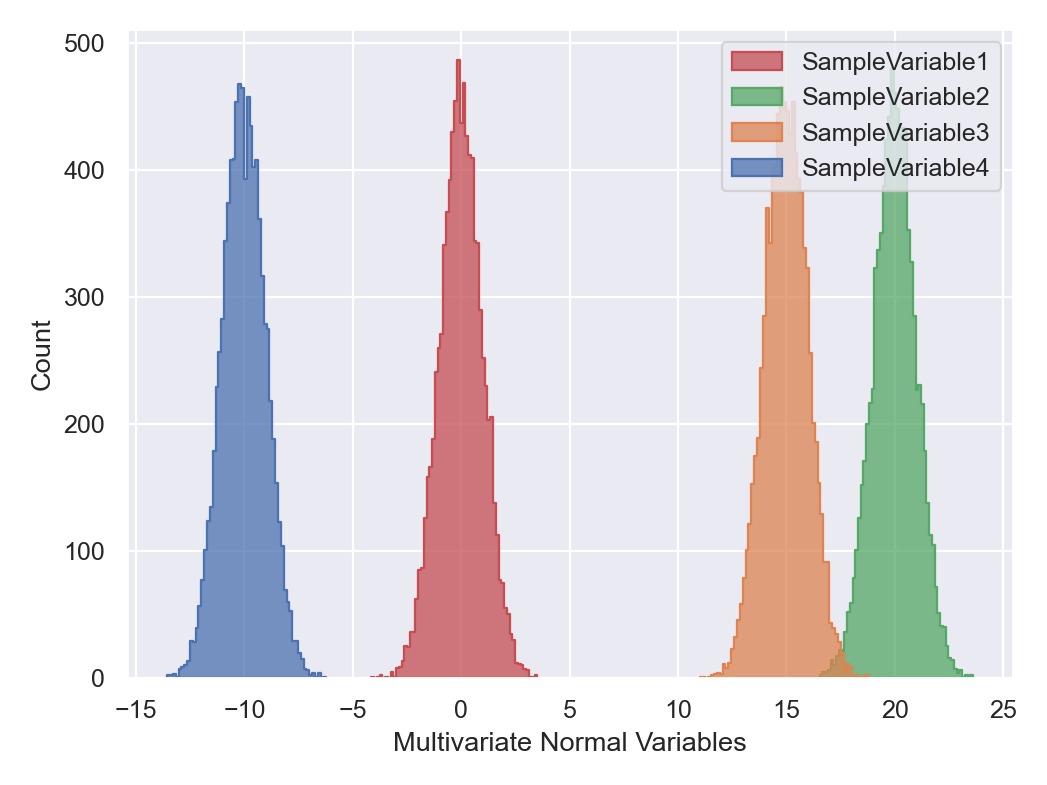done in 0.20597 seconds.

Out:
Text(0.5, 31.07499999999998, 'Multivariate Normal Variables')

To make a trace-plot of the sample, try,

In :
sample.plot.line()

ParaDRAM - NOTE: making the line plot...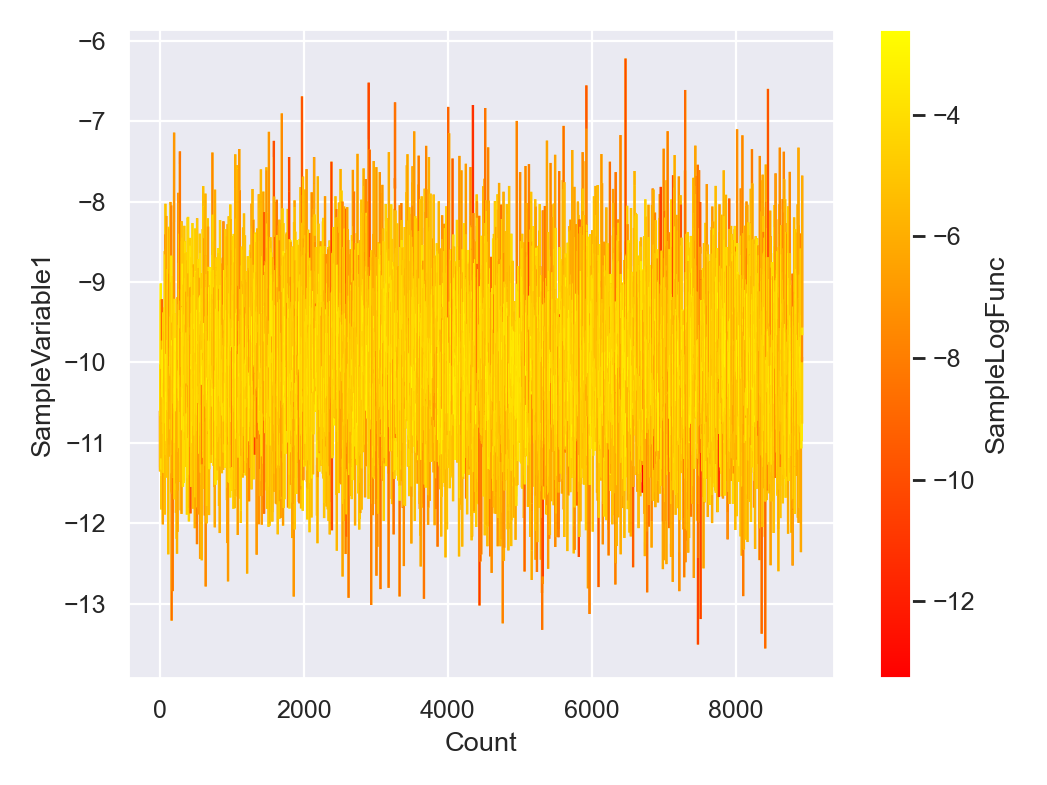done in 0.286038 seconds.


To change the scale of the x-axis, try,

In :
sample.plot.line()
sample.plot.line.currentFig.axes.set_xscale("log")

ParaDRAM - NOTE: making the line plot...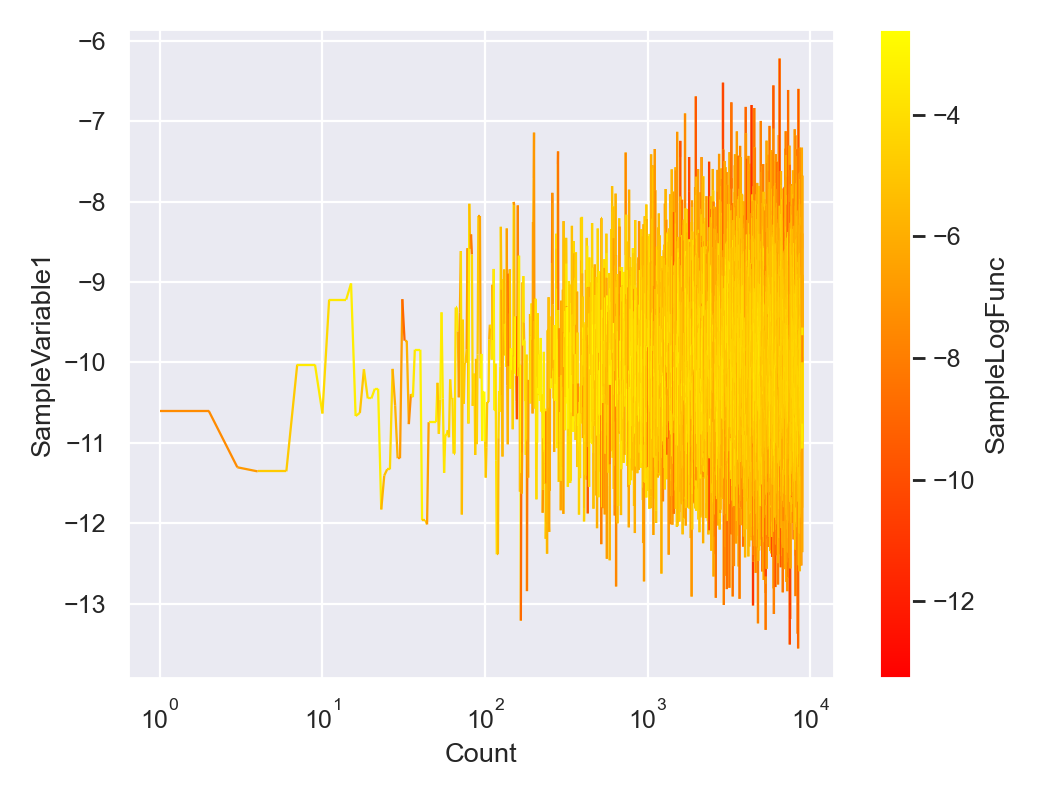done in 0.218998 seconds.


By default, the color of the line in the trace-plot will represent the value returned by getLogFunc() at the given sampled point.

In :
sample.plot.line.ccolumns

Out:
'SampleLogFunc'

To turn the color off, we can instead try,

In :
sample.plot.line.ccolumns = None
sample.plot.line()
sample.plot.line.currentFig.axes.set_xscale("log")

ParaDRAM - NOTE: making the line plot...